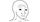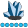somebody
Lapis Lazuli | Level 10

## How does PROC ARIMA know what time/date variable?

I am trying to get a cross-correlogram between stock returns and trading volumes and run across PROC ARIMA. I wonder how does PROC ARIMA know which variable is the time variable? In PROC TIMESERIES or PROC EXPAND, we have to specify a time variable with an ID statement, and if there are many groups (in my case, stocks), we have to specify a BY statement.

Does PROC ARIMA assume there are only 1 group and the data is already sorted by time sequence?

1 ACCEPTED SOLUTION

Accepted Solutions

## Re: How does PROC ARIMA know what time/date variable?

PROC ARIMA assumes the data are equally-spaced and sorted sequentially over time, therefore, a time ID variable is not required to compute the cross-correlations.  If you have gaps in the data, then these gaps should be filled in with missing values (or some other value of your choosing) prior to running the procedure in order to preserve the correct spacing between observations.

PROC ARIMA supports a BY statement so you can perform a separate analysis for each BY group.  Within each BY group, PROC ARIMA assumes the data is equally-spaced and sorted sequentially over time.

I hope this helps!

DW

3 REPLIES 3Ksharp
Super User

## Re: How does PROC ARIMA know what time/date variable?

Please post it at Forecasting Forum.

## Re: How does PROC ARIMA know what time/date variable?

I moved this to the Forecasting community.

## Re: How does PROC ARIMA know what time/date variable?

PROC ARIMA assumes the data are equally-spaced and sorted sequentially over time, therefore, a time ID variable is not required to compute the cross-correlations.  If you have gaps in the data, then these gaps should be filled in with missing values (or some other value of your choosing) prior to running the procedure in order to preserve the correct spacing between observations.

PROC ARIMA supports a BY statement so you can perform a separate analysis for each BY group.  Within each BY group, PROC ARIMA assumes the data is equally-spaced and sorted sequentially over time.

I hope this helps!

DW

Discussion stats
• 3 replies
• 393 views
• 1 like
• 4 in conversation